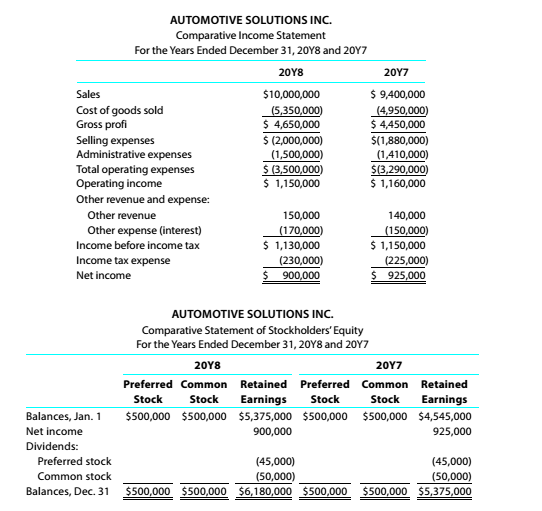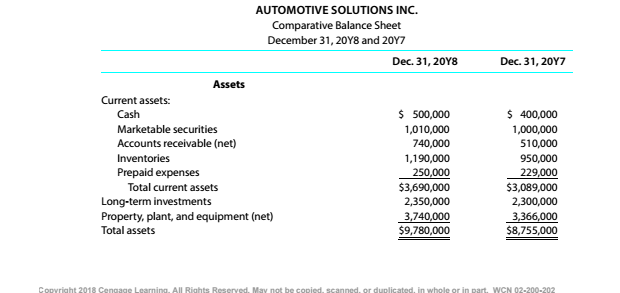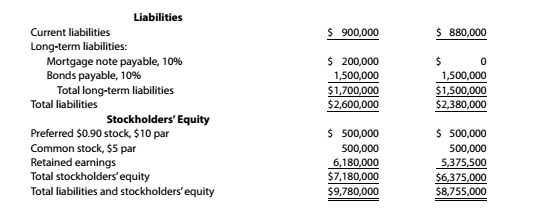Chapter 9, Problem 9.4.15P

Chapter
Section
Textbook Problem

Twenty metrics of liquidity, solvency, and profitabilityThe comparative financial statements of Automotive Solutions Inc. are as follows. The market price of Automotive Solutions Inc. common stock was $119.70 on December 31, 20Y8InstructionsReturn on stockholders’ equity To determine Concept Introduction: Return on Equity: Return on Equity is the rate of return earned by Stockholders on investment in a company. It is calculated with the help of following formula: Return on Equity = Net IncomeAverage Stockholders Equity The Average stock holder's equity calculated with the help of following formula: Average stock holders equity=( Beginning stock holders equity + Ending stock holders equity)2 To Calculate: The Return on Stockholder's Equity Explanation The Return on Stockholder's Equity is calculated as follows:  20Y8 Net Income (A)$ 900,000 Beginning Stockholder's Equity (B) \$ 6,375,000

Still sussing out bartleby?

Check out a sample textbook solution.

See a sample solution

The Solution to Your Study Problems

Bartleby provides explanations to thousands of textbook problems written by our experts, many with advanced degrees!

Get Started

Find more solutions based on key concepts